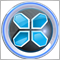# Indicator not sending value to EA32

I'm trying to develop a simple indicator that tells me the difference between 2 similar products eg. UK & US Oil. The indicator correctly displays variance on a chart but does not send the same value to my EA.

Any help would be appreciated.

```//+------------------------------------------------------------------+
//|                                                       OilSpread2.mq4 |
//|                                   |
//+------------------------------------------------------------------+

#property indicator_chart_window

extern color font_color = Red;
extern int font_size = 14;
extern string font_face = "Arial";
extern int corner = 0; //0 - for top-left corner, 1 - top-right, 2 - bottom-left, 3 - bottom-right
extern int spread_distance_x = 10;
extern int spread_distance_y = 130;
extern bool normalize = false; //If true then the spread is normalized to traditional pips

double Poin;
int n_digits = 0;
double divider = 1;

//+------------------------------------------------------------------+
//| Custom indicator initialization function                         |
//+------------------------------------------------------------------+
int init()
{
//Checking for unconvetional Point digits number
if (Point == 0.00001) Poin = 0.0001; //5 digits
else if (Point == 0.001) Poin = 0.01; //3 digits
else Poin = Point; //Normal

ObjectCreate("Spread", OBJ_LABEL, 0, 0, 0);
ObjectSet("Spread", OBJPROP_CORNER, corner);
ObjectSet("Spread", OBJPROP_XDISTANCE, spread_distance_x);
ObjectSet("Spread", OBJPROP_YDISTANCE, spread_distance_y);
double spread = MarketInfo("XBRUSD",MODE_ASK) - MarketInfo("XTIUSD",MODE_BID);

if ((Poin > Point) && (normalize))
{
divider = 10.0;
n_digits = 1;
}

ObjectSetText("Spread", "Spread: " + DoubleToStr(NormalizeDouble(spread / divider, 1), n_digits) + " points.", font_size, font_face, font_color);

return(0);
}

//+------------------------------------------------------------------+
//| Custom indicator deinitialization function                       |
//+------------------------------------------------------------------+
int deinit()
{
ObjectDelete("Spread");
return(0);
}

//+------------------------------------------------------------------+
//| Custom indicator iteration function                              |
//+------------------------------------------------------------------+
int start()
{
RefreshRates();

double spread = (MarketInfo("XBRUSD",MODE_ASK) - MarketInfo("XTIUSD",MODE_BID)) / Point;
ObjectSetText("Spread", "Spread: " + DoubleToStr(NormalizeDouble(spread / divider, 1), n_digits) + " points.", font_size, font_face, font_color);

return(0);
}
//+------------------------------------------------------------------+```28587

Hi,

So you didnt implement a buffer for it,you should define a buffer,then spend the variance value into the buffer,or you could do it via global variables.

Or you could do this formula directly into your EA:

```double spread = (MarketInfo("XBRUSD",MODE_ASK) - MarketInfo("XTIUSD",MODE_BID)) / Point/divider;
```32

Mehrdad Jeddi:

Hi,

So you didnt implement a buffer for it,you should define a buffer,then spend the variance value into the buffer,or you could do it via global variables.

Or you could do this formula directly into your EA:

I put the code as suggested into the EA & disabled the indicator. EA now works fine.  Many thanks Mehrdad.28587

wsandy59:

I put the code as suggested into the EA & disabled the indicator. EA now works fine.  Many thanks Mehrdad.

OK,Good,You're welcome,

To add comments, please log in or register